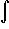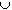#Interactive Real Analysis

Next | Previous | Glossary | Map

## 7.4. Lebesgue Integral

### Proposition 7.4.10: Properties of the Lebesgue Integral

Suppose f and g are two bounded, Lebesgue integrable functions defined on a measurable set E with finite measure. Then:
1.E c f(x) + d g(x) dx = cE f(x) dx + dE g(x) dx
2. If A and B are disjoint measurable subsets of E thenAB f(x) dx =A f(x) dx +B f(x) dx
3. If f(x) = g(x) for all x in E except possibly on a set of measure zero thenE f(x) dx =E g(x) dx
4. If f(x)g(x) for all x in E except possibly on a set of measure zero thenE f(x) dxE g(x) dx
Next | Previous | Glossary | Map